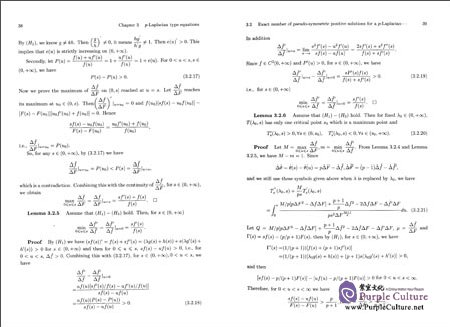FEEDBACK

#### Solvable Theory of Nonlinear Differential Equations and Their Applications

Price: \$42.45 \$29.83 (Save \$12.62)
Only 3 left in stock, order soon.

Author: Zhang Xuemei;
Language: English
Format: 24.2 x 17 x 2.2 cm
Page: 327
Publication Date: 03/2016
ISBN: 9787030472304
Publisher: Science Press
##### Details

<br/>
<b>Editor's Recommendation</b>
<p>《非线性微分方程的可解性理论及其应用(英文版)》由北京教育科学出版社出版。</p>
<br/>

Chapter 1 Progress in Nonlinear Differential Equations
1.1 p—Laplacian type equations
1.2 Prescribed mean curvature equations
1.3 Impulsive differential equations
1.4 Functional differential equations
1.5 Fractional differential equations
1.6 Elastic beam equations
References
Chapter 2 Basic Definitions and Theorems
2.1 Cone and Fixed point theorems
2.2 Coincidence degree theory
2.3 Fractional calculus
2.4 Holder's Inequality
2.5 Time—map Analysis and Bifurcation Diagrams
References
Chapter 3 p—Laplacian type equations
3.1 Exact number of solutions for a class of two point boundary value problems with one—dimensional p—Laplacian
3.2 Exact number of pseudo—symmetric positive solutions for a p—Laplacian three—point boundary value problems
3.3 Existence of a positive solution for one—dimensional singular p—Laplacian problems and its parameter dependence
References
Chapter 4 Prescribed Mean Curvature Operator Equations
4.1 Exact multiplicity and bifurcation diagrams of positive soiutions of a one—dimensional prescribed mean curvature equation with concave—convex nonlinearities
4.2 Time map analysis to establish the exact number of positive solutions of one—dimensional prescribed mean curvature equations
4.3 Periodic solutions for prescribed mean curvature Lienard equation with a deviating argument
References
Chapter 5 Green's Function and Fractional Differential Equations
5.1 The existence of positive solution to a nonlinear fractional differential equation with integral boundary conditions
5.2 New existence results for higher—order nonlinear fractional differential equation with integral boundary conditions
5.3 Green's function for Sturm—Liouville—type boundary value problems of fractional order impulsive differential equations and its application
References
Chapter 6 Elasticity Beam Equations
6.1 Symmetric positive solutions of elasticity beam equations with integral boundary conditions
6.2 New existence theory of positive solutions to fourth order p—Laplacian elasticity problems
6.3 Multiple positive solutions of fourth order impulsive differential equations with integral boundary conditions and one—dimensionai p—L aplacian
References
Chapter 7 Functional Differential Equations
7.1 Existence， multiplicity， and dependence on a parameter for a functional differential equation
7.2 Positive Deriodic solutions of first—order impulsive functional differential equations
7.3 Periodic solution and nontrivial periodic solutions for a class of Rayleigh type equation with two deviating arguments
7.4 Anti—periodic solutions to Rayleigh—type equations with two deviating arguments
7.5 Positive solutions for a second—order singular differential equations with a delayed argument
7.6 Positive solutions for second order impulsive p—Laplacian equations with deviating arguments
References
Chapter 8 Nonlinear Impulsive Differential Equations
8.1 Parameter dependence of positive solutions for second order singular impulsive differential equations
8.2 Impulsive differential equations of one—dimensional singular p—Laplacian with two parameters
8.3 Multi—parameter fourth order impulsive differential equations witn one—dimensional m—Laplacian and deviating arguments
8.4 Transformation techniques and fixed point theories to establish the positive solutions of second order impulsive differential equations
8.5 Transformation technique and positive solutions for second—order impulsive differential equations with deviating arguments